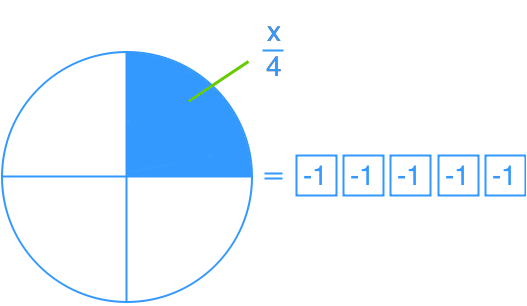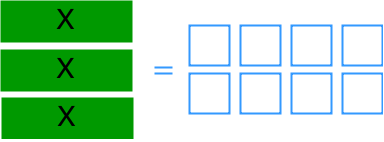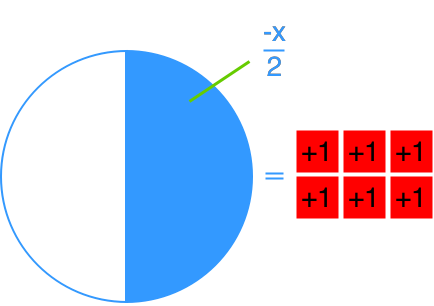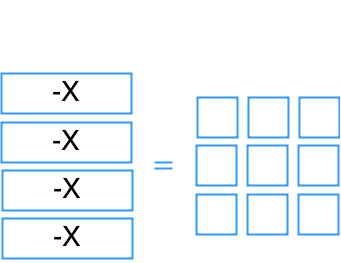# Model and solve one-step linear equations: ax = b, x/a = b#### Everything You Need in One Place

Homework problems? Exam preparation? Trying to grasp a concept or just brushing up the basics? Our extensive help & practice library have got you covered.#### Learn and Practice With Ease

Our proven video lessons ease you through problems quickly, and you get tonnes of friendly practice on questions that trip students up on tests and finals.#### Instant and Unlimited Help

Our personalized learning platform enables you to instantly find the exact walkthrough to your specific type of question. Activate unlimited help now!

0/5
##### Intros
###### Lessons
1. What is Reflexive property of equality?
2. What is Transitive property of equality?
3. What is Substitution property of equality?
4. What is Symmetric property of equality?
5. How to solve one-step equations?
0/10
##### Examples
###### Lessons
1. What is the equation represented by each diagram?

1.2.3.4.2. Solve.
1. $- 4x = 56$
2. $3x = - 24$
3. $\frac{x}{5} = - 75$
4. $- 2 = \frac{x}{{ - 10}}$
3. Bill is able to type 35 words every minute. If he has typed 385 words so far, how many minutes has he been typing for?
1. Suzy and 2 friends split their restaurant bill evenly. Each person paid \$16. How much did the bill cost?
0%
##### Practice
###### Topic Notes
Linear equations which can be solved with a single operation are called one-step linear equations. In this section, we will try to solve one-step linear equations represented in diagrams and in equation form.